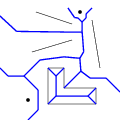CGAL 5.0 - L Infinity Segment Delaunay Graphs
L Infinity Segment Delaunay Graphs ReferencePanagiotis Cheilaris, Sandeep Kumar Dey, Evanthia Papadopoulou
Algorithms and geometric traits for computing the dual of the Voronoi diagram of a set of points and segments under the $$L_{\infty}$$ metric.
Introduced in: CGAL 4.7
Depends on: 2D Segment Delaunay Graphs
BibTeX: cgal:cdp-sdglinf2-19b

CGAL provides algorithms for computing the segment Delaunay graph, i.e., the dual of the Voronoi diagram of segments, under the $$L_{\infty}$$ metric. The algorithms are implemented in the classes CGAL::Segment_Delaunay_graph_Linf_2<Gt> and CGAL::Segment_Delaunay_graph_Linf_hierarchy_2<Gt>. The template parameter Gt must be a model of the SegmentDelaunayGraphLinfTraits_2 concept, which is related to the geometric objects and predicates associated with computing segment Delaunay graphs, under the $$L_{\infty}$$ distance. The classes CGAL::Segment_Delaunay_graph_Linf_traits_2<K,MTag>, CGAL::Segment_Delaunay_graph_Linf_traits_without_intersections_2<K,MTag> CGAL::Segment_Delaunay_graph_Linf_filtered_traits_2<K,MTag> CGAL::Segment_Delaunay_graph_Linf_filtered_traits_without_intersections_2<K,MTag> are models of the SegmentDelaunayGraphLinfTraits_2 concept.

Concepts

Classes

class  CGAL::Segment_Delaunay_graph_Linf_2< Gt, DS >
The class Segment_Delaunay_graph_Linf_2 represents the segment Delaunay graph under the $$L_{\infty}$$ metric (which is the dual graph of the 2D segment Voronoi diagram under the $$L_{\infty}$$ metric). More...

struct  CGAL::Segment_Delaunay_graph_Linf_filtered_traits_2< CK, CM, EK, EM, FK, FM >
The class Segment_Delaunay_graph_Linf_filtered_traits_2 provides a model for the SegmentDelaunayGraphLinfTraits_2 concept. More...

struct  CGAL::Segment_Delaunay_graph_Linf_filtered_traits_without_intersections_2< CK, CM, EK, EM, FK, FM >
The class Segment_Delaunay_graph_Linf_filtered_traits_without_intersections_2 provides a model for the SegmentDelaunayGraphLinfTraits_2 concept. More...

class  CGAL::Segment_Delaunay_graph_Linf_hierarchy_2< Gt, STag, DS >
This class is equivalent to the Segment_Delaunay_graph_hierarchy_2 class, but it uses Segment_Delaunay_graph_Linf_2<Gt,DS> at every level of the hierarchy (instead of Segment_Delaunay_graph_2<Gt,DS> at each level of Segment_Delaunay_graph_hierarchy_2). More...

struct  CGAL::Segment_Delaunay_graph_Linf_traits_2< K, MTag >
The class Segment_Delaunay_graph_Linf_traits_2 provides a model for the SegmentDelaunayGraphLinfTraits_2 concept. More...

struct  CGAL::Segment_Delaunay_graph_Linf_traits_without_intersections_2< K, MTag >
The class Segment_Delaunay_graph_Linf_traits_without_intersections_2 provides a model for the SegmentDelaunayGraphLinfTraits_2 concept. More...